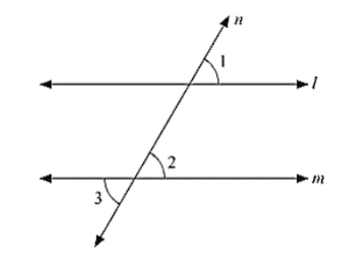# In the below fig, ∠1 = 60° and ∠2 = (2/3)rd of a right angle. Prove that l ∥ m.

Question:

In the below fig, ∠1 = 60° and ∠2 = (2/3)rd of a right angle. Prove that l ∥ m.Solution:

Given:

∠1 = 60° and ∠2 = (2/3)rd of a right angle

To Prove: Parallel Drawn to m

Proof ∠1 = 60

∠2 = (2/3) × 90 = 60

Since ∠1 = ∠1 = 60°

Therefore, Parallel to m as pair of corresponding angles are equal.